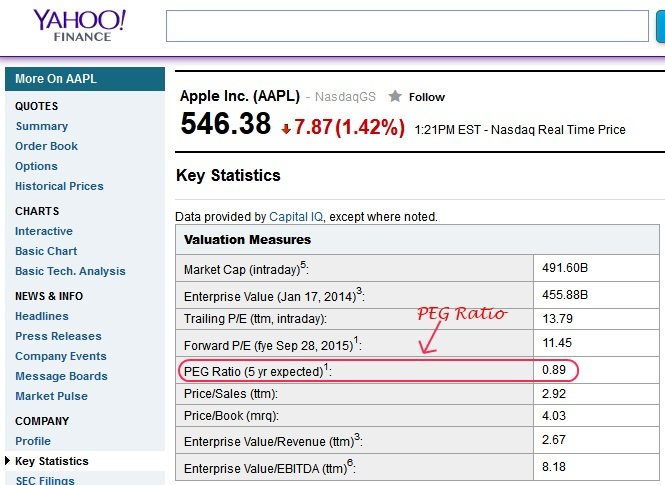Select Page

# PEG Ratio – Price/Earnings To Growth RatioThe Price/Earnings To Growth ratio (PEG ratio) is similar to the PE Ratio, but it takes it one step further by accounting for future earnings growth.

The PEG ratio is used to determine a stock’s value while factoring in the company’s earnings growth.

Some consider the PEG ratio to provide a more complete picture than the P/E ratio. While a high P/E ratio might make a stock look overvalued, factoring in the company’s growth rate to get the stock’s PEG ratio can tell a different story.

## How The PEG Ratio Formula is Calculated

The PEG ratio calculation uses the basic format of the P/E ratio for a numerator and then divides it by the estimated annual EPS growth rate.

Calculated as: (P/E Ratio) / Projected Annual Growth in Earnings per Share = PEG RatioBefore you are able to find the PEG ratio, you must first know what the price to earnings ratio (p/e ratio) of the stock is. Once the P/E ratio is known, you simply divide that by the estimated annual EPS growth rate.

The growth rate is expressed as a percentage and some investors calculate it based on the growth rate for the next 12 months, while others might use the estimated next five years’ worth.

## PEG Ratio Example

For example, if a company has a P/E of 25 and is growing at 30% a year, it would have a PEG ratio of .83
(25 ÷ 30 = .83).

Lets take a look at Apple Inc. (NASDAQ:AAPL) and find out the PEG ratio. Some financial websites display the PEG ratio so you don’t have to figure out the peg ratio calculation yourself, such as Yahoo Finance, which uses 5 yr expected growth rate.As you can see Apple currently has a PEG ratio of 0.89 using a 5 yr expected growth rate.

## What Is A Good PEG Ratio

Typically, a PEG ratio of less than 1.0 implies that the stock is undervalued and the market is not fully valuing the prospects for future growth. A ratio over 1.0 might imply that the stock is reaching overvalued stage.

The downside of the PEG ratio is that future growth rates are sometimes hard to predict. Some companies’ growth profiles can change considerably over time.